# Introduction to reda by Examples

#### 2016-12-15

The package reda mainly provides function to fit gamma frailty model with either a piecewise constant or a spline as the baseline rate function for recurrent event data. What’s more, some handy functions are designed, such as computing and plotting sample nonparametric mean cumulative function, or so-called Nelson-Aalen estimator. Most functions in this package are S4 methods that produce S4 class objects.

In this vignette, we mainly introduce the basic usage of the functions provided in the package by examples. The details of function syntax and slots of the objects produced are available in the package manual, which will thus not be covered in this vignette.

An outline of the remainder of the vignette is as follows: We first introduce the simulated sample recurrent event data and the data checking rules. After then, the demonstration of the main function rateReg for model fitting is provided, which includes fitting model with (one piece) constant, piecewise constant, and spline baseline rate function. What follows next are the examples of functions that summarize the model fitted and functions for model selection based on AIC or BIC. Last but not the least, the demonstration of estimation and plotting methods for baseline rate function and mean cumulative function (MCF) are given, which includes sample MCF and estimated MCF from the fitted model.

## Simulated Sample Recurrent Event Data

library(reda)

First of all, the sample recurrent event data we are going to use in the following examples is called simuDat, which contains totally 500 observations of 6 variables.

head(simuDat)
##   ID time event group    x1 gender
## 1  1    1     1 Contr -1.93 female
## 2  1   22     1 Contr -1.93 female
## 3  1   23     1 Contr -1.93 female
## 4  1   57     1 Contr -1.93 female
## 5  1  112     0 Contr -1.93 female
## 6  2  140     0 Treat -0.11 female
str(simuDat)
## 'data.frame':    500 obs. of  6 variables:
##  $ID : num 1 1 1 1 1 2 3 3 4 4 ... ##$ time  : num  1 22 23 57 112 140 40 168 14 112 ...
##  $event : int 1 1 1 1 0 0 1 0 1 0 ... ##$ group : Factor w/ 2 levels "Contr","Treat": 1 1 1 1 1 2 1 1 1 1 ...
##  $x1 : num -1.93 -1.93 -1.93 -1.93 -1.93 -0.11 0.2 0.2 -0.43 -0.43 ... ##$ gender: Factor w/ 2 levels "female","male": 1 1 1 1 1 1 1 1 1 1 ...

where

• ID: Subjects identification.
• time: Event or censoring time.
• event: Event indicator, 1 = event; 0 = censored.
• group: Treatment group indicator.
• x1: Continuous variable.
• gender: Gender of subjects.

The dataset is originally simulated by thinning method (Lewis and Shedler 1979) and further processed for a better demonstration purpose.

## Data Checking

In the main function rateReg for model fitting, formula response is specified by function Survr, which has a considerate data checking procedure embedded for recurrent event data modeled by method based on counts and rate function. Therefore, before model fitting, the observations of the covariates specified in the formula will be checked. The checking rules include

• Identification of each subject cannot be missing.
• Event indicator must be coded as 0 (censored) or 1 (event).
• Event time and censoring time cannot be missing.
• Each subject must have one and only one censoring time.
• Event time cannot not be later than censoring time.

The subject’s ID will be pinpointed if its observation violates any checking rule shown above.

## Model Fitting

### Model with Constant Rate Function

The default model when argument df, knots, and degree are not specified is gamma frailty model with (one piece) constant rate function, which is equivalent to negative binomial regression with the same shape and rate parameter in the gamma prior.

(constFit <- rateReg(Survr(ID, time, event) ~ group + x1, data = simuDat))
## Call:
## rateReg(formula = Survr(ID, time, event) ~ group + x1, data = simuDat)
##
## Coefficients of covariates:
## groupTreat         x1
## -0.6133735  0.3248214
##
## Frailty parameter:  0.5877632
##
## Boundary knots:
## 0, 168
##
## Coefficients of pieces:
##  B-spline1
## 0.03049925

The function rateReg returns rateReg-class object, which can be printed out by calling the object. (Internally, show method for rateReg object is called.)

### Model with Piecewise Constant Rate Function

When argument df or knots (at least one internal knot) is specified, the model becomes gamma frailty model with piecewise constant rate function or so-called HEART model (Fu, Luo, and Qu 2016) if argument degree is specified to be zero as default.

We may specify df and leave knots and degree as default. Then piecewise constant rate function will be applied and the number of pieces will equal df. The internal knots will be automatically specified at suitable quantiles of the covariate representing event and censoring time.

For example, two pieces’ constant rate function can be simply specified by setting df = 2. The internal knot will be the median time of all the event and censoring time. Also, we can fit the models on the first 50 subjects by specifying argument subset.

# two pieces' constant rate function
(twoPiecesFit <- rateReg(Survr(ID, time, event) ~ group + x1, df = 2,
data = simuDat, subset = ID %in% 1:50))
## Call:
## rateReg(formula = Survr(ID, time, event) ~ group + x1, data = simuDat,
##     subset = ID %in% 1:50, df = 2)
##
## Coefficients of covariates:
## groupTreat         x1
## -0.7884974  0.3388589
##
## Frailty parameter:  0.6917535
##
## Internal knots:
## 102
##
## Boundary knots:
## 0, 168
##
## Coefficients of pieces:
##  B-spline1  B-spline2
## 0.03396700 0.04889329

In the example shown above, the internal knots is set automatically to be 102 and the baseline rate function is two pieces’ constant.

If internal knots are specified explicitly, the df will be neglected even if it is specified. An example of model with six pieces’ constant rate function is given as follows:

## df = 2 will be neglected since knots are explicitly specified
(piecesFit <- rateReg(Survr(ID, time, event) ~ group + x1, data = simuDat,
df = 2, knots = seq(from = 28, to = 140, by = 28)))
## Call:
## rateReg(formula = Survr(ID, time, event) ~ group + x1, data = simuDat,
##     df = 2, knots = seq(from = 28, to = 140, by = 28))
##
## Coefficients of covariates:
## groupTreat         x1
## -0.6374185  0.3062430
##
## Frailty parameter:  0.5868408
##
## Internal knots:
## 28, 56, 84, 112, 140
##
## Boundary knots:
## 0, 168
##
## Coefficients of pieces:
##  B-spline1  B-spline2  B-spline3  B-spline4  B-spline5  B-spline6
## 0.02576637 0.03049018 0.02061309 0.03057382 0.03771134 0.04788783

### Model with Spline Rate Function

When argument degree is specified to be a positive integer, the baseline rate function is fitted by splines. The type or flavor of the splines can be specified by argument spline. The available option for spline are bSplines for B-splines and mSplines for M-splines. (See R package spline2 for details about the spline functions used internally.) A partial matching on names is allowed.

For example, one may want to fit the baseline rate function by a cubic spline with two internal knots. Then we may explicitly specify degree = 3 and knots to be a length-two numeric vector. Or we may simply specify degree = 3 and df = 6 Then the internal knots will be automatically specified at suitable quantiles of the covariate representing event and censoring time. Generally speaking, the degree of freedom of spline (or the number of spline bases) equals the summation of the number of internal knots and the degree of each spline base, plus one if intercept is included in spline bases.

## internal knots are set as 33% and 67% quantiles of time variable
(splineFit <- rateReg(Survr(ID, time, event) ~ group + x1, data = simuDat,
df = 6, degree = 3, spline = "mSplines"))
## Call:
## rateReg(formula = Survr(ID, time, event) ~ group + x1, data = simuDat,
##     df = 6, degree = 3, spline = "mSplines")
##
## Coefficients of covariates:
## groupTreat         x1
## -0.6360341  0.3057453
##
## Frailty parameter:  0.5882718
##
## Internal knots:
## 73.33333, 138
##
## Boundary knots:
## 0, 168
##
## Coefficients of spline bases:
## M-spline1 M-spline2 M-spline3 M-spline4 M-spline5 M-spline6
## 0.3431309 1.5541666 0.1479342 1.7828600 1.3690646 0.1828824
## or internal knots are expicitly specified
(splineFit <- rateReg(Survr(ID, time, event) ~ group + x1, data = simuDat,
spline = "bSplines", degree = 3L, knots = c(56, 112)))
## Call:
## rateReg(formula = Survr(ID, time, event) ~ group + x1, data = simuDat,
##     knots = c(56, 112), degree = 3L, spline = "bSplines")
##
## Coefficients of covariates:
## groupTreat         x1
## -0.6355965  0.3061800
##
## Frailty parameter:  0.5884861
##
## Internal knots:
## 56, 112
##
## Boundary knots:
## 0, 168
##
## Coefficients of spline bases:
##  B-spline1  B-spline2  B-spline3  B-spline4  B-spline5  B-spline6
## 0.01809729 0.04107795 0.01771056 0.02400282 0.06319184 0.03174401

## Summary of Model Fits

A brief summary of the fitted model is given by show method as shown in the previous examples. Further, summary method for rateReg-class object provides a more specific summary of the model fitted. For instance, the summary of the models fitted in section of model fitting can be called as follows:

summary(constFit)
## Call:
## rateReg(formula = Survr(ID, time, event) ~ group + x1, data = simuDat)
##
## Coefficients of covariates:
##                coef exp(coef) se(coef)       z Pr(>|z|)
## groupTreat -0.61337   0.54152  0.28601 -2.1446  0.03199 *
## x1          0.32482   1.38378  0.16642  1.9518  0.05096 .
## ---
## Signif. codes:  0 '***' 0.001 '**' 0.01 '*' 0.05 '.' 0.1 ' ' 1
##
## Parameter of frailty:
##         parameter        se
## Frailty 0.5877632 0.1102639
##
## Boundary knots:
## 0, 168
##
## Degree of spline bases: 0
##
## Coefficients of spline bases:
##               coef se(coef)
## B-spline1 0.030499   0.0058
##
## Loglikelihood:  -1676.421
summary(piecesFit, showCall = FALSE)
## Coefficients of covariates:
##                coef exp(coef) se(coef)       z Pr(>|z|)
## groupTreat -0.63742   0.52866  0.29095 -2.1908  0.02847 *
## x1          0.30624   1.35831  0.16698  1.8340  0.06665 .
## ---
## Signif. codes:  0 '***' 0.001 '**' 0.01 '*' 0.05 '.' 0.1 ' ' 1
##
## Parameter of frailty:
##         parameter       se
## Frailty 0.5868408 0.109938
##
## Internal knots:
## 28, 56, 84, 112, 140
##
## Boundary knots:
## 0, 168
##
## Degree of spline bases: 0
##
## Coefficients of spline bases:
##               coef se(coef)
## B-spline1 0.025766   0.0059
## B-spline2 0.030490   0.0068
## B-spline3 0.020613   0.0049
## B-spline4 0.030574   0.0069
## B-spline5 0.037711   0.0084
## B-spline6 0.047888   0.0108
##
## Loglikelihood:  -1663.792
summary(splineFit, showCall = FALSE, showKnots = FALSE)
## Coefficients of covariates:
##                coef exp(coef) se(coef)       z Pr(>|z|)
## groupTreat -0.63560   0.52962  0.28713 -2.2136  0.02685 *
## x1          0.30618   1.35823  0.16653  1.8385  0.06598 .
## ---
## Signif. codes:  0 '***' 0.001 '**' 0.01 '*' 0.05 '.' 0.1 ' ' 1
##
## Parameter of frailty:
##         parameter        se
## Frailty 0.5884861 0.1103426
##
## Degree of spline bases: 3
##
## Coefficients of spline bases:
##               coef se(coef)
## B-spline1 0.018097   0.0076
## B-spline2 0.041078   0.0117
## B-spline3 0.017711   0.0100
## B-spline4 0.024003   0.0109
## B-spline5 0.063192   0.0168
## B-spline6 0.031744   0.0131
##
## Loglikelihood:  -1663.285

The summary includes the function call, estimated covariate coefficients, estimated parameter of frailty variable, internal knots (if exist), boundary knots, degree of spline bases if splines are applied, coefficients of rate function bases (pieces), and log-likelihood of the model fitted. Outputs of function call or knots, may be suppressed by specifying argument showCall or showKnots to be FALSE, respectively, in summary method, which would be especially useful for a relatively concise summary in a reproducible report using Rmarkdown, etc.

What’s more, the corresponding coef and confint method for point estimates and confidence interval for covariate coefficients are provided as well. Let’s take the fitted model with spline rate function as an example.

## point estimates of covariate coefficients
coef(splineFit)
## groupTreat         x1
## -0.6355965  0.3061800
## confidence interval for covariate coefficients
confint(splineFit, level = 0.95)
##                   2.5%       97.5%
## groupTreat -1.19835943 -0.07283366
## x1         -0.02022021  0.63258029

## Model Selection

Two handy functions are provided for model selection. We may compare and select the models with different baseline rate function based on Akaike Information Criterion (AIC) by function AIC or Bayesian Information Criterion (BIC) by function BIC.

A friendly warning will be thrown out if the numbers of observation were different in the model comparison by AIC.

AIC(constFit, piecesFit, splineFit)
##           df      AIC
## constFit   4 3360.843
## piecesFit  9 3345.585
## splineFit  9 3344.570
BIC(constFit, piecesFit, splineFit)
##           df      BIC
## constFit   4 3377.701
## piecesFit  9 3383.516
## splineFit  9 3382.501

## Baseline Rate Function

Function baseRate produces baseRateReg-class object representing the estimated baseline rate function for a fitter model. An associated plot method is available. For example, the baseline rate function and its confidence band estimated by cubic splines can be plotted as follows:

baseRateObj <- baseRate(splineFit)
plot(baseRateObj, conf.int = TRUE)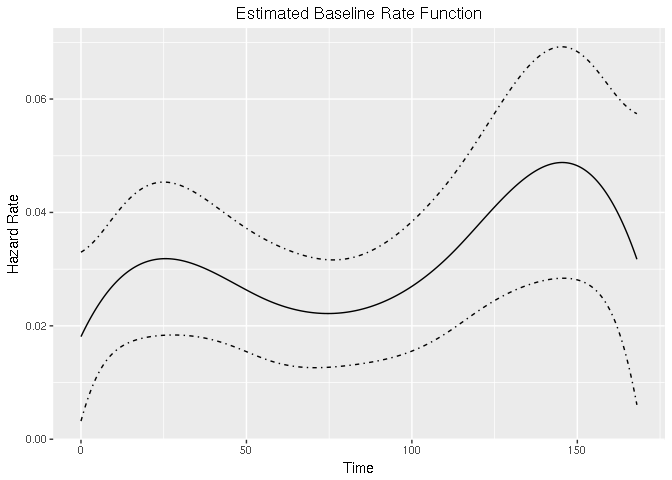## Mean Cumulative Function (MCF)

The generic function computing the sample MCF and the estimated MCF from the fitted model is called mcf. The related methods are dispatched by function plot for plotting the estimated MCF by using ggplot2 plotting system.

### Sample MCF (Nelson-Aalen Estimator)

The nonparametric sample MCF is also called Nelson-Aalen Estimator (Nelson 2003). The point estimate of MCF at each time point does not assume any particular underlying model. The variance estimates at each time point is given by Poisson process method (by default) or Lawless and Nadeau method (Lawless and Nadeau 1995). The approximate confidence intervals are provided as well, which are constructed based on the asymptotic normality of logarithm of MCF (by default) or MCF itself directly. ReliaWiki (2012) also provided a simple example.

If a formula with Survr as response is specified in function mcf, the method for sample MCF will be called. The covariate specified at the right hand side of the formula should be either 1 or any “linear” combination of factor variables in the data. The former computes the overall sample MCF. The latter computes the sample MCF for each level of the combination of the factor variable(s) specified, respectively.

The valve-seat dataset in Nelson (1995) and the sample simulated data are used for demonstration as follows:

## overall sample MCF for valve-seat data in Nelson (1995)
valveMcf <- mcf(Survr(ID, Days, No.) ~ 1, data = valveSeats)

## sample MCF for different groups
simuMcf <- mcf(Survr(ID, time, event) ~ group + gender,
data = simuDat, subset = ID %in% 1 : 50, logConfInt = FALSE)

After estimation, we may plot the sample MCF by function plot, which actually returns a ggplot object so that the plot produced can be easily further customized by functions in package ggplot2. The legendname and legendLevels can be used to customize the legend in the plot. Examples are given as follows:

## Example 1. valve-seat data
plot(valveMcf, conf.int = TRUE, mark.time = TRUE) + ggplot2::xlab("Days")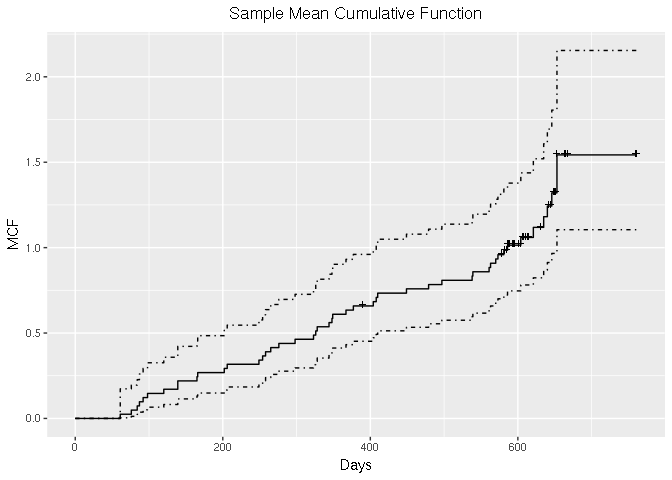## Example 2. sample simulated data
## plot after creating customized levels in legend
levs <- with(simuDat, expand.grid(levels(group), levels(gender)))
levs <- do.call(paste, c(as.list(levs), sep = " & "))
plot(simuMcf, conf.int = TRUE, lty = 1 : 4, legendName = "Treatment & Gender",
legendLevels = levs) + ggplot2::xlab("Days")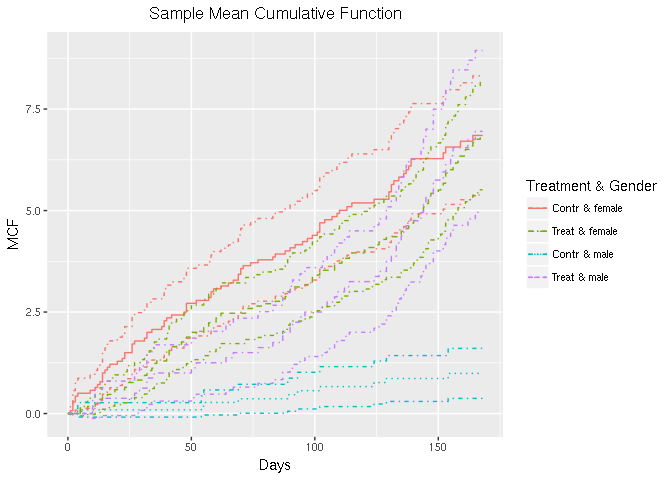Note that all the censoring time can be marked on the step curve by specifying mark.time = TRUE. The type and color of the line can be specified through lty and col, respectively.

### Estimated MCF from the fitted Model

If rateReg-class object is supplied to function mcf, the method for rateReg-class is called, which returns the estimated baseline MCF from the fitted model if newdata is not specified in the function.

The example estimating and plotting the baseline MCF from the fitted model with piecewise constant rate function is shown as follows:

piecesMcf <- mcf(piecesFit)
plot(piecesMcf, conf.int = TRUE, col = "blueviolet") + ggplot2::xlab("Days")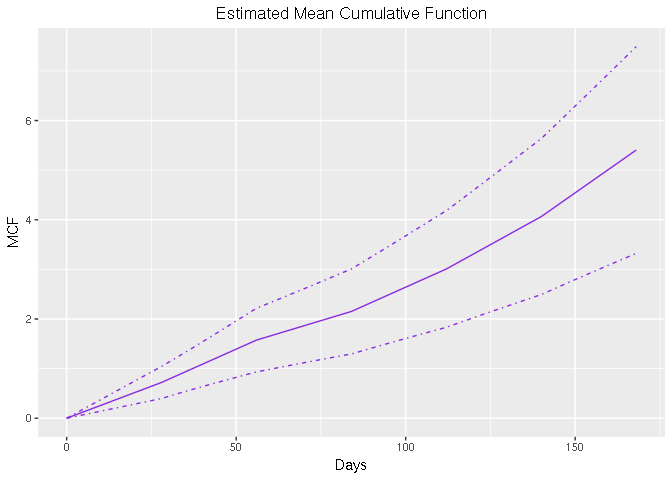The argument newdata allows one to estimate the MCF for a given dataset instead of the baseline MCF. If newdata is specified, the data frame should have the same column names as the covariate names appearing in the formula of original fitting. The MCF will be estimated for each unique row in the data frame and its confidence intervals are constructed based on Delta-method.

In addition, we may specify the name for grouping each unique row and the levels of each group through groupName and groupLevels, respectively. For example, we may specify groupName = "Gender" and groupLevels = c("Male", "Female") for estimation of different gender groups.

As the last example in this vignette, we estimate the MCF from fitted model with spline rate function for the different treatment groups and plot the estimated MCFs and their confidence intervals correspondingly.

newDat <- data.frame(x1 = rep(0, 2), group = c("Treat", "Contr"))
estmcf <- mcf(splineFit, newdata = newDat, groupName = "Group",
groupLevels = c("Treatment", "Control"))
plot(estmcf, conf.int = TRUE, col = c("royalblue", "red"), lty = c(1, 5)) +
ggplot2::ggtitle("Control vs. Treatment") + ggplot2::xlab("Days")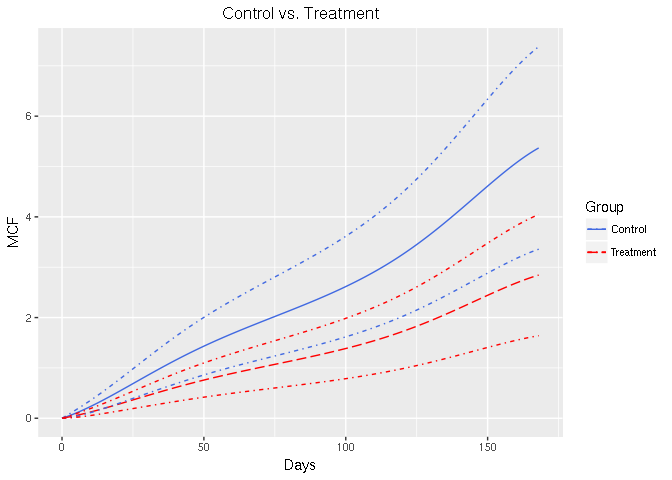## Reference

Fu, Haoda, Junxiang Luo, and Yongming Qu. 2016. “Hypoglycemic Events Analysis via Recurrent Time-to-Event (HEART) Models.” Journal of Biopharmaceutical Statistics 26 (2): 280–98. doi:10.1080/10543406.2014.992524.

Lawless, Jerald F, and Claude Nadeau. 1995. “Some Simple Robust Methods for the Analysis of Recurrent Events.” Technometrics 37 (2). Taylor & Francis: 158–68.

Lewis, P. A., and G. S. Shedler. 1979. “Simulation of Nonhomogeneous Poisson Processes by Thinning.” Naval Research Logistics Quarterly 26 (3). Wiley Online Library: 403–13.

Nelson, Wayne B. 1995. “Confidence Limits for Recurrence Data—Applied to Cost or Number of Product Repairs.” Technometrics 37 (2). Taylor & Francis: 147–57.

———. 2003. Recurrent Events Data Analysis for Product Repairs, Disease Recurrences, and Other Applications. Vol. 10. SIAM.

ReliaWiki. 2012. “Recurrent Event Data Analysis.” http://reliawiki.org/index.php/Recurrent_Event_Data_Analysis.